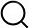# Also in the Article

Complete melting threshold
This protocol is extracted from research article:
Visualization of ultrafast melting initiated from radiation-driven defects in solids

Procedure

The complete melting threshold is the energy that would be sufficient to completely melt the film. This complete melting threshold can be expressed in terms of absorbed pump fluence, Fm, and is given by (30)$Fm=(∫300TmCl(T)dT+∫300TmCe(T)dT+ΔHm)L$(3)where L is the thin film thickness, Tm is the nominal melting temperature, and ΔHm is the latent heat. Equation 3 implies that the complete melting threshold is the amount of energy that is required to heat the material from room temperature to its equilibrium melting temperature and to melt the entire film at the melting temperature. Using the following parameters: ΔHm = 280 kJ/kg (31), Tm = 3695 K (31), and taking into account the temperature dependence of Cl(T) (38) and Ce(T) (39), we estimated Fm to be approximately 52 mJ/cm2 for 30-nm-thick W film.

Note: The content above has been extracted from a research article, so it may not display correctly.

Q&A